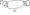# VTU Previous Year Exam Papers EC 3rd Sem Logic design January 2008

Note : Answer any FIVE full questions choosing at least TWO questions from each part.

PART-A

1 a. Using Karnaugh map simplify the following Boolean expression and give the implementation of the same using :

i) NAND gates only (SOP form) ii) NOR gates only (POS form)

f (A, B, C*B).= ]Tm (0., 1, 2S 4, 5, 12, 14) + do (8, 10).

b.  Find the prime implicantes for the Boolean expression using Quine Me dusky5s method. F(w,X,Y,Z)=£m(l,3,6,7,8,9,10,12, 13, 14).

c.   Explain the principle of duality.

2 a. Realize the Boolean expression f(w, x, y, z) = ^m (4, 6, 7, 8, 10, 12s 15) using a 4 to 1 line multiplexer and external gates.

b.  Design a 1-bit comparator using basic gates.

c.   Implement the following Boolean functions using an appropriate PLA.

F1(A,B,C)= m(0,4,7) ; F2 (A, B, C) = £m(4,6).

d.  What are the three different models for writing a module body in Verilog HDL. Give an example for any one model.

3 a.Explain with example the 2ss complement arithmetic using all the cases.

b.  Draw a block diagram of a 4 – bit adder – subtract circuit using full adder and give a brief description.

c.   Design a 2-bit fast adder. Give its implementation using gates.

4 a. Write a HDL code for a full adder. (04 Marks)

a.   Write the characteristic of an ideal clock.

b.  With the help of a block diagram, explain the working of a JK Master – Slave flip – flop.

c.   Show how a SR flip ~ flop can be converted to a JK flip – flop.

PART-B

5  a. Distinguish between a ring counter and a Johnson counter.

b.  Explain the working of a 3-bit asynchronous down counter.

c.   Design a synchronous mod – 5 up counter using JK flip – flop. Give excitation table of JK flip – flop, state diagram and state table.

6  a. Explain the difference between Mealy and Moore models.

b.  Reduce the state transition diagram by row elimination method and implication table method.c.   Design an asynchronous sequential logic circuit for the state transition diagram shown.7 a. Draw a 4-bit D/A converter mmg R/2R resistors and explam its working.

b. Explain the A/D converter by simultaneous conversion. Draw the block diagram of a 2 – bit simultaneous A/D converter.

8 a. With the aid of a circuit diagram, explain the operation of a 2 – input TTL NAND gate with totem – pole output

b.  Explain the operation of a 2 – input CMOS NOR gate with a help of a circuit diagram.

c.   Write a note on the CMOS characteristics.